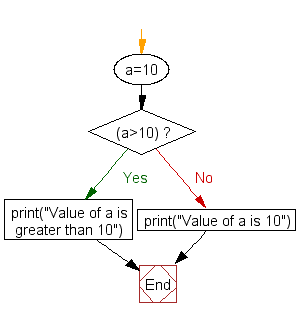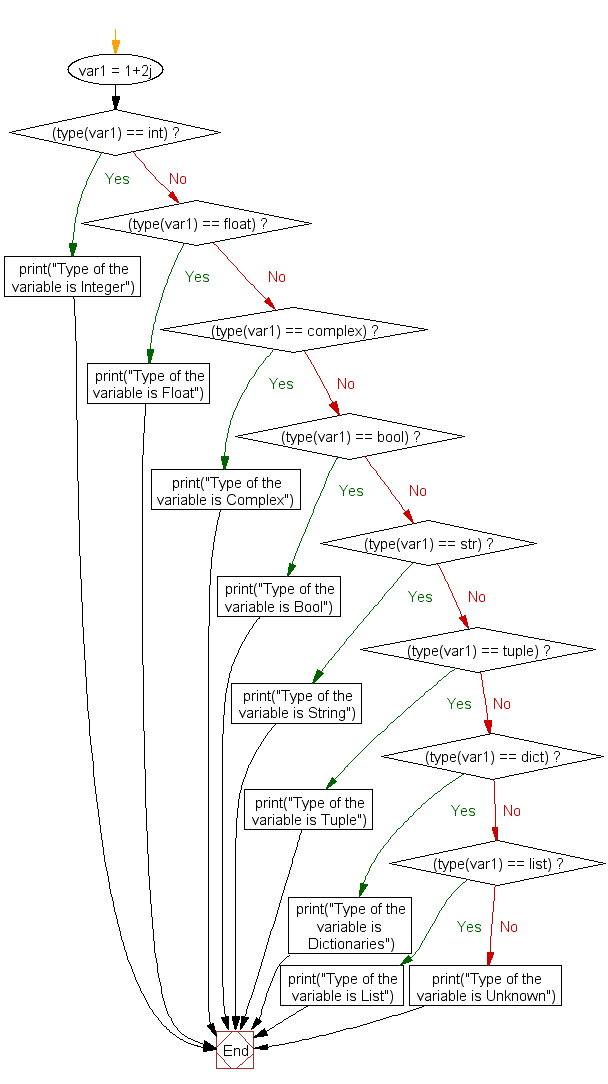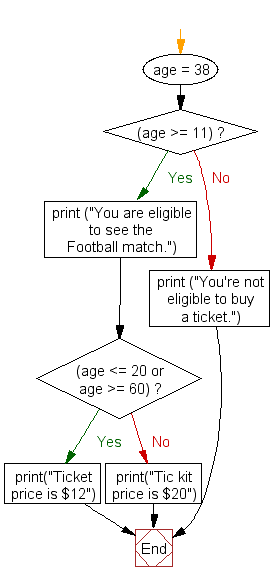# Python if elif else

## if..elif..else

The if-elif-else statement is used to conditionally execute a statement or a block of statements. Conditions can be true or false, execute one thing when the condition is true, something else when the condition is false.

## if statement

The Python if statement is same as it is with other programming languages. It executes a set of statements conditionally, based on the value of a logical expression.

Here is the general form of a one way if statement.

Syntax:

```if expression :
statement_1
statement_2
....
```

In the above case, expression specifies the conditions which are based on Boolean expression. When a Boolean expression is evaluated it produces either a value of true or false. If the expression evaluates true the same amount of indented statement(s) following if will be executed. This group of the statement(s) is called a block.

## if .. else statement

In Python if .. else statement, if has two blocks, one following the expression and other following the else clause. Here is the syntax.

Syntax:

```
if expression :
statement_1
statement_2
....
else :
statement_3
statement_4
....
```

In the above case if the expression evaluates to true the same amount of indented statements(s) following if will be executed and if the expression evaluates to false the same amount of indented statements(s) following else will be executed. See the following example. The program will print the second print statement as the value of a is 10.

``````a=10
if(a>10):
print("Value of a is greater than 10")
else :
print("Value of a is 10")
``````

Output:

`Value of a is 10`

Flowchart:## if .. elif .. else statement

Sometimes a situation arises when there are several conditions. To handle the situation Python allows adding any number of elif clause after an if and before an else clause. Here is the syntax.

Syntax:

```
if expression1 :
statement_1
statement_2
....

elif expression2 :
statement_3
statement_4
....
elif expression3 :
statement_5
statement_6
....................
else :
statement_7
statement_8
```

In the above case Python evaluates each expression (i.e. the condition) one by one and if a true condition is found the statement(s) block under that expression will be executed. If no true condition is found the statement(s) block under else will be executed. In the following example, we have applied if, series of elif and else to get the type of a variable.

``````var1 = 1+2j
if (type(var1) == int):
print("Type of the variable is Integer")
elif (type(var1) == float):
print("Type of the variable is Float")
elif (type(var1) == complex):
print("Type of the variable is Complex")
elif (type(var1) == bool):
print("Type of the variable is Bool")
elif (type(var1) == str):
print("Type of the variable is String")
elif (type(var1) == tuple):
print("Type of the variable is Tuple")
elif (type(var1) == dict):
print("Type of the variable is Dictionaries")
elif (type(var1) == list):
print("Type of the variable is List")
else:
print("Type of the variable is Unknown")
``````

Output:

`Type of the variable is Complex`

Flowchart:## Nested if .. else statement

In general nested if-else statement is used when we want to check more than one conditions. Conditions are executed from top to bottom and check each condition whether it evaluates to true or not. If a true condition is found the statement(s) block associated with the condition executes otherwise it goes to next condition. Here is the syntax :

Syntax:

```
if expression1 :
if expression2 :
statement_3
statement_4
....
else :
statement_5
statement_6
....
else :
statement_7
statement_8
```

In the above syntax expression1 is checked first, if it evaluates to true then the program control goes to next if - else part otherwise it goes to the last else statement and executes statement_7, statement_8 etc.. Within the if - else if expression2 evaluates true then statement_3, statement_4 will execute otherwise statement_5, statement_6 will execute. See the following example.

``````age = 38
if (age >= 11):
print ("You are eligible to see the Football match.")
if (age <= 20 or age >= 60):
print("Ticket price is \$12")
else:
print("Tic kit price is \$20")
else:
print ("You're not eligible to buy a ticket.")
``````

Output :

```You are eligible to see the Football match.
Tic kit price is \$20
```

In the above example age is set to 38, therefore the first expression (age >= 11) evaluates to True and the associated print statement prints the string "You are eligible to see the Football match". There after program control goes to next if statement and the condition ( 38 is outside <=20 or >=60) is matched and prints "Tic kit price is \$12".

Flowchart:## Use the and operator in an if statement

``````#create two boolean objects

x = False
y = True

#The validation will be True only if all the expressions generate a value True
if x and y:
print('Both x and y are True')
else:
print('x is False or y is False or both x and y are False')
``````

Output:

`x is False or y is False or both x and y are False`

## Use the in operator in an if statement

``````#create a string
s = 'jQuery'
#create a list
l = ['JavaScript', 'jQuery', 'ZinoUI']

# in operator is used to replace various expressions that use the or operator
if s in l:
print(s + ' Tutorial')

#Alternate if statement with or operator

if s == 'JavaScript' or s == 'jQuery' or s == 'ZinoUI':
print(s + ' Tutorial')
``````

Output:

```jQuery Tutorial
jQuery Tutorial
```

## Write an if-else in a single line of code

``````#create a integer
n = 150
print(n)

#if n is greater than 500, n is multiplied by 7, otherwise n is divided by 7
result = n * 7 if n > 500 else n / 7
print(result)
``````

Output:

```150
21.428571428571427
```

## Define a negative if

If a condition is true the not operator is used to reverse the logical state, then logical not operator will make it false.

``````#create a integer
x = 20
print(x)

#uses the not operator to reverse the result of the logical expression

if not x == 50:
print('the value of x different from 50')
else:
print('the value of x is equal to 50')
``````

Output:

``` 20
the value of x different from 50
```

Test your Python skills with w3resource's quiz

﻿

## Python: Tips of the Day

Getting min/max from iterable (with/without specific function):

```# Getting maximum from iterable
>>> a = [1, 2, -3]
>>> max(a)
2

# Getting maximum from iterable
>>> min(a)
1

# Bot min/max has key value to allow to get maximum by appliing function
>>> max(a,key=abs)
3
```